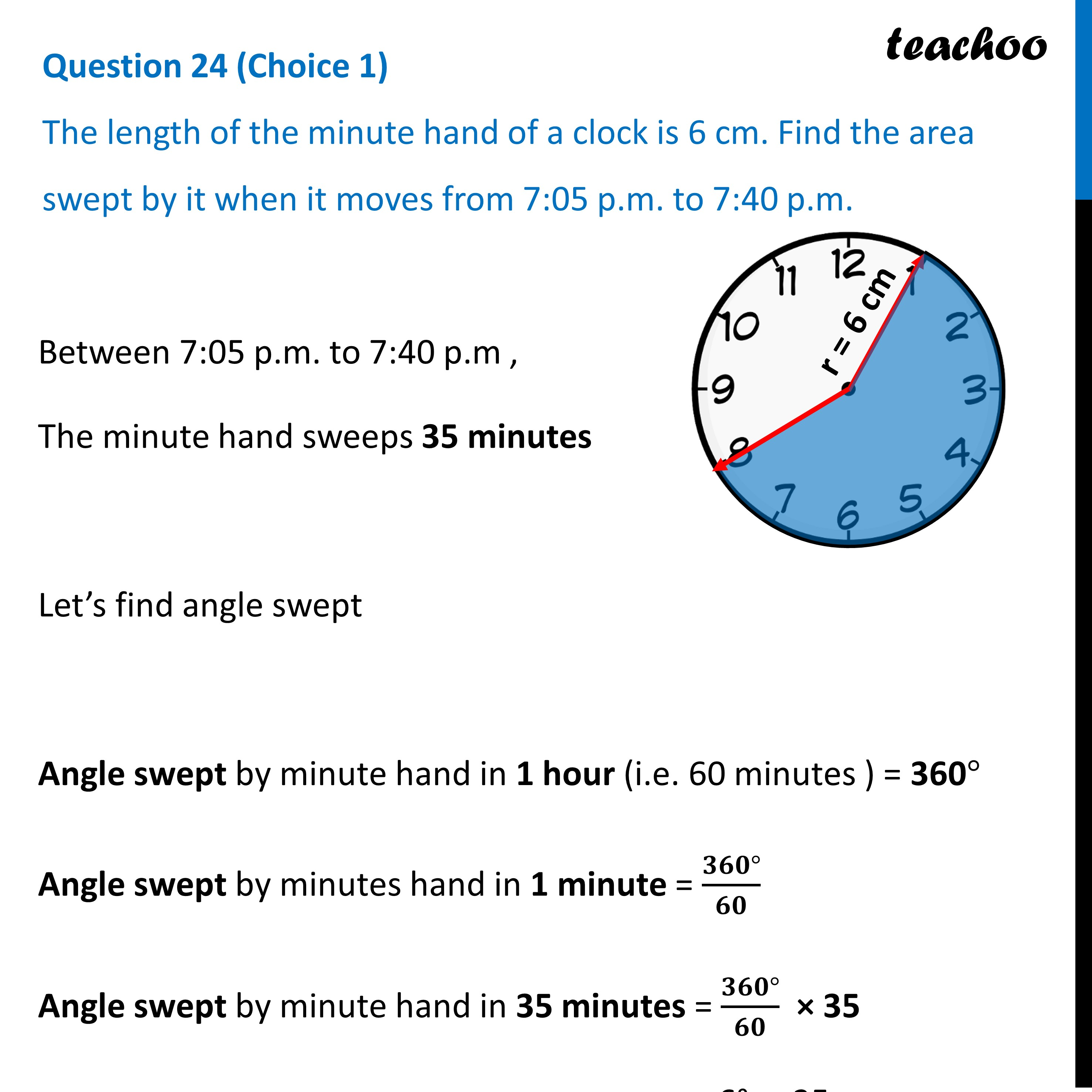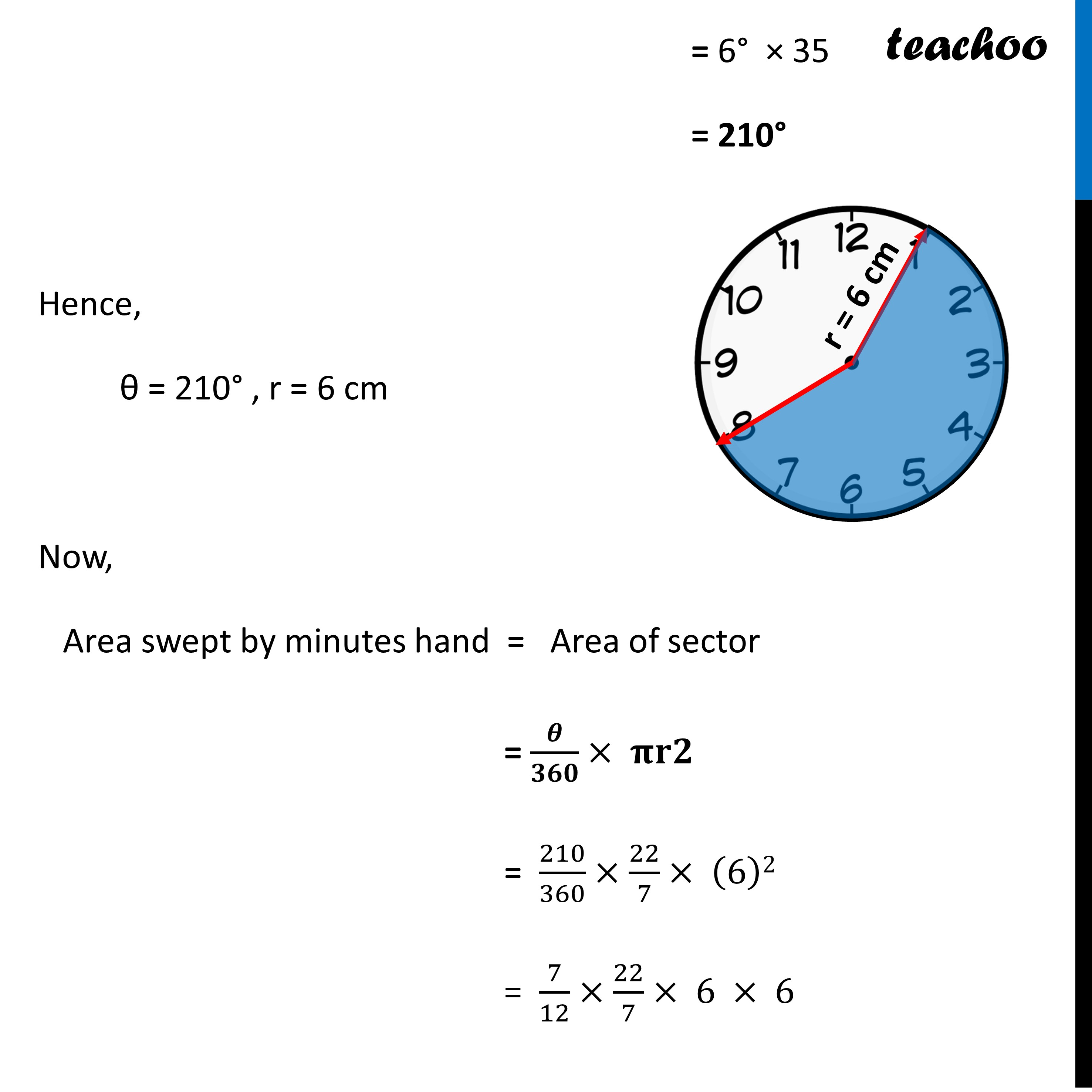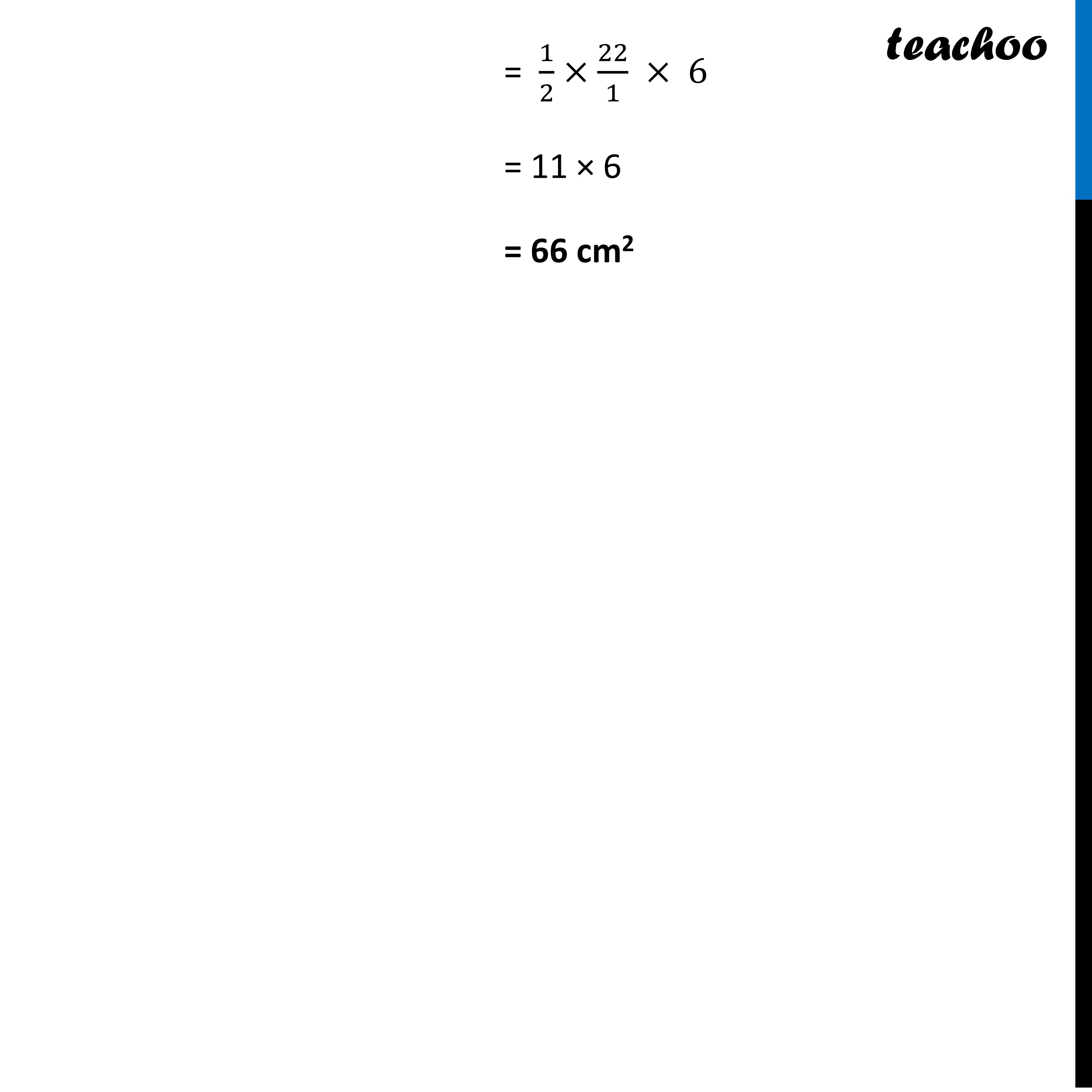CBSE Class 10 Sample Paper for 2023 Boards - Maths Standard

Class 10
Solutions of Sample Papers for Class 10 Boards

## The length of the minute hand of a clock is 6 cm. Find the area swept by it when it moves from 7:05 p.m. to 7:40 p.m.

This question is Similar to Question Ex-12.2-3 - Chapter 12 Class 10Learn in your speed, with individual attention - Teachoo Maths 1-on-1 Class

### Transcript

Question 24 (Choice 1) The length of the minute hand of a clock is 6 cm. Find the area swept by it when it moves from 7:05 p.m. to 7:40 p.m. Between 7:05 p.m. to 7:40 p.m , The minute hand sweeps 35 minutes Let’s find angle swept Angle swept by minute hand in 1 hour (i.e. 60 minutes ) = 360° Angle swept by minutes hand in 1 minute = (𝟑𝟔𝟎°)/𝟔𝟎 Angle swept by minute hand in 35 minutes = (𝟑𝟔𝟎°)/𝟔𝟎 × 35 = 6° × 35 = 210° Hence, θ = 3210° , r = 6 cm Now, Area swept by minutes hand = Area of sector = θ/360×πr2 = 210/360×22/7×(14)2 = 1/2×22/1 × 6 = 11 × 6 = 66 cm2